## Introduction

Ultralong-range Rydberg molecules, theoretically predicted in ref. 1, show very weak binding energies, similar to magneto-associated Feshbach molecules2,3, and thus require ultracold temperatures. The bond in these molecules results from the scattering of a slow Rydberg electron from a neutral atom with a negative scattering length a (ref. 1). The theoretical approach is based on Fermi’s original concept of the pseudopotential4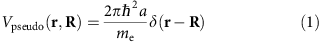describing such a scattering event between an electron of mass me at position r and an atom at R. If the scattering length a is negative, the interaction is attractive and ground state atoms can be bound in a potential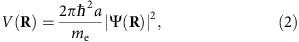where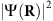is the local electron density. In case of 87Rb the theoretical value of the scattering length a for triplet scattering is −16.1a0 (ref. 5), where a0 is the Bohr radius. The singlet scattering length is positive and therefore does not lead to a bound state.

The vibrational ground state wavefunction belonging to the diatomic (polyatomic) molecules with the highest binding energy per atom is localized mainly at the position of the outermost lobe of the electron wavefunction, that is, near the classical turning point of the electron. Molecules containing more than one ground state atom can be described with the same formalism. Two ground state atoms inside the electron orbit essentially do not interact with each other, as the Rb atom–atom scattering length6 is much smaller than the mean particle distance in a dilute thermal cloud. Hence, the binding energy of an i-atomic molecule, iN, is (i–1) times larger than the binding energy of a dimer. Only when the number of ground state atoms inside the Rydberg atom becomes very large, a change in the description of the system from discrete bound states to a mean field approximation is required. In this case no individual bound states are resolved, but the Rydberg line is shifted by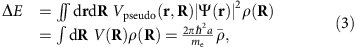where ρ is the ground state atom density and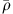its average weighted with the probability density of the Rydberg electron. If higher order corrections to the zero-energy scattering length can be neglected, the mean shift depends only on the value of a and.

A wide range of fascinating phenomena, starting from the coherent creation and breaking of chemical bonds7 to a permanent electric dipole moment in a homonuclear molecule8, have been already studied in the field of ultralong-range Rydberg molecules. Dimers, consisting of a single atom in the Rydberg state and one atom in the ground state, have been observed in an ultracold gas of Rb in the Rydberg S-state9, D-state10,11 and P-state12, and of Cs (ref. 13) in the Rydberg S-state. The regime, where the Rydberg electron experiences a mean shift by thousands of atoms within its orbit, has been studied at very high densities in a Bose–Einstein condensate, leading to electron–phonon coupling14, and in a hot vapour15. Here we trace the transition between the resolvable molecular lines and the mean shift regime in an ultracold cloud with a constant density by tuning only one parameter: the principal quantum number n of the excited Rydberg state. We probe the limit at which the binding energy per atom becomes very small, while the radius of the molecules grows beyond 10,000a0.

## Results

### Molecular spectroscopy

In Fig. 1 excitation spectra from the 5S1/2 to the nS1/2 state, where n is ranging from 51 to 111, are presented. Comparison of some of the relevant molecular potentials are shown in Fig. 2. The shape of the obtained Rydberg spectra varies significantly for different n. For low principal quantum numbers clearly distinguishable molecular lines are present on the red side of the atomic peak, which is situated at the origin. We can identify the observed molecular lines by calculating the binding energies of dimers in the ground and the first vibrationally excited state9. The binding energy EB can be directly measured as the difference between the atomic and the molecular line in the spectrum. In the spectrum of n=51 the peak at −1.7 MHz can be identified as a dimer, for which the ground state atom is localized in the outermost well of the molecular potential. Additionally, at −1.3 MHz an excited vibrational state9,16 is visible. For a given density, the probability to find an atom inside the electron orbit scales as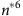with the effective principal quantum number n*=nδ0. Hence, higher order molecules are formed more likely at higher n. At the same time the binding energy per atom decreases and thus polyatomic molecular lines become visible in the spectra of n=62 and 71. At n=62, lines up to the tetramer together with corresponding vibrational excited states are visible. The broadening of the tetramer line may be caused by the presence of these excited states, possibly with a reduced lifetime17. At n=71 only vibrational ground states are resolved. Polyatomic molecules up to a pentamer can be identified. The size of such a molecule becomes enormous due to the Rydberg electron orbit radius reaching almost 10,000a0 (Fig. 1). Since the thermal cloud is much bigger than the Rydberg atom, the Gaussian density distribution does not play a role on a single Rydberg atom level, and the spatial arrangement of the bound ground state atoms is isotropic. For large n the binding energy EB decreases until it is below the experimental resolution. This manifests in a non-resolvable shoulder and finally in an inhomogeneously broadened spectral line.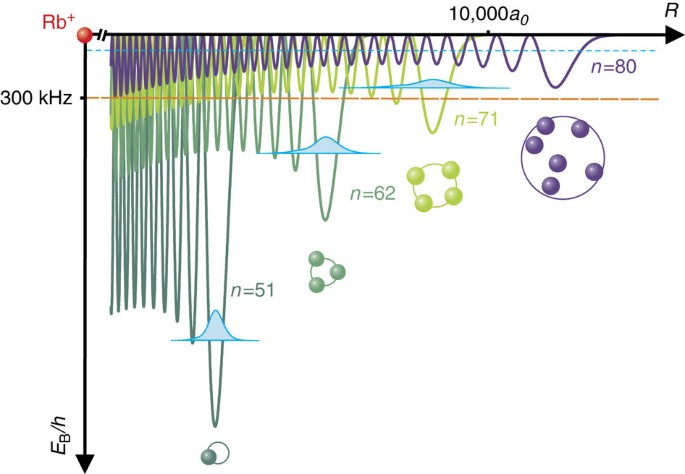Figure 2: Scattering potentials for n=51, 62, 71 and 80 S-states.

### Analysis of the spectra

The experimental binding energies can be calculated based on the molecular potential (2) and the mean shift (3). Momentum-dependent corrections to these values can be estimated using a semiclassical approximation18. Additionally, a p-wave shape resonance can cause a substantial modification of the molecular potential, leading to butterfly-type molecules19 and states bound by internal quantum reflection16. These corrections are largely negligible for high principal quantum numbers and large distances from the Rydberg core, where the relative motion of the Rydberg electron and the perturber is slow. We use corrections to the s-wave scattering length including terms linear in the relative momentum. As this approximation is valid for large distances from the ionic core, we restrict the analysis to the lowest bound state. The discussion of higher vibrational states can be found in ref. 16. We solve the Schrödinger equation for the ground state atom in the molecular potential using Numerov’s method to find the binding energies of the dimers. We fit the zero-energy scattering length a to obtain the best agreement with the measured values. In this paper we achieve the best agreement with the experimental data for −16.2a0, which is very close to the theoretically predicted value of −16.1a0 (ref. 5).

For n>71, where no distinct molecular lines can be identified, a mean field description is required. Furthermore, the spectral position of pure Rydberg atoms, and thus the zero position, cannot be identified directly from the signal. However it can be determined from the centre of gravity cg of the spectra, taken in a non-blockaded sample, which is constant for a given density. Intuitively, this result in the first approximation can be explained by the fact that while the mean potential depth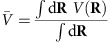averaged over the volume of the Rydberg atom decreases with the effective principal number as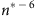, the probability to find an atom inside the Rydberg electron orbit increases with. In the experiment all data were taken at a fixed density. Thus, in our analysis we overlap the centre of gravity of the spectra at n≥71 with the mean 〈cg〉=−300 kHz determined at low principal quantum numbers. Doing so we can identify the zero position in the top panels of Fig. 1. Assuming the scattering length a to be constant, we determine the effective density to be 3 × 1012 cm−3, which is close to the peak density obtained from a Gaussian fit to absorption images of the thermal cloud. This indicates that molecules are most likely created in regions of high density. Note that the power broadening would not effect the centre of gravity of the measured spectra. Only for n≤80 the highest signal originates from pure Rydberg atoms. Already for n=80 there is on average one ground state atom inside the electron orbit, leading to a high probability to excite dimers instead of pure Rydberg atoms. On average there are four atoms inside the 100S electron orbit and eight for the 111S state. Therefore, the atomic line is suppressed, while the molecular contribution to the overall signal increases. At the same time the molecular lines are not resolvable in the experiment any more, because of their very low binding energies. For even higher principal quantum numbers the shape of the spectrum is expected to become Gaussian, with the maximum at the position of the mean shift (〈cg〉 in Fig. 1) (refs 14, 20).

The binding energies of all observed molecules are plotted in Fig. 3. From the extrapolated molecular binding energies the transition from the few particle description of discrete bound states to a mean field shift becomes visible. A power law fitted to the n≥40 data shows a scaling with the effective principal quantum number n* to the power of −6.26±0.12, close to the value of −6, expected from the size scaling argument. The deviation can be explained by the dependence of the scattering length on the relative momentum and the fact that with increasing principal quantum number the shape of the outermost well of the molecular potential changes. Taking the corresponding zero-point energy and momentum-dependent corrections to the scattering length into account, we obtain an exponent of −6.37, which is in very good agreement with our experimental data. Contributions of higher order partial waves and p-wave shape resonances to the molecular potential can be neglected, as the kinetic energy associated with the relative motion of two scattering partners is small in the region of interest.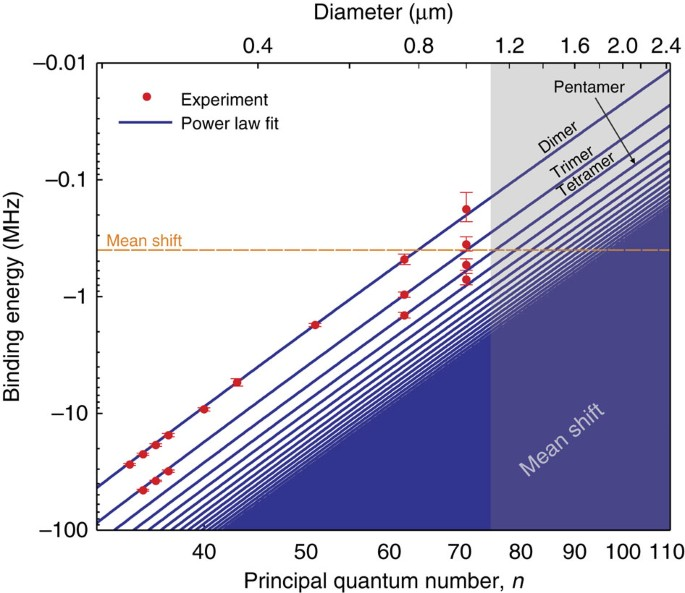Figure 3: Measured binding energies versus principal quantum number and diameters of the molecules.

## Discussion

The obtained experimental results have strong implications, for example, in the field of Rydberg quantum optics. The density-dependent dephasing resulting from the existence of many molecular lines within the Rydberg line envelope sets a fundamental limit for the number of atoms inside the blockade radius, that is, the optical thickness, for n>80. This fact is of importance for every experiment taken at high principal quantum numbers and high densities, in particular for quantum optics experiments in ultracold clouds21,22,23,24. In many experiments based on electromagnetically induced transparency using a Rydberg state, the figure of merit is high optical depth per Rydberg blockade volume, which is set by the width of the electromagnetically induced transparency window25. Assuming a typical linewidth of 1 MHz, density-induced decoherence becomes relevant at the density 1013 cm−3 (ref. 23). Therefore, this effect has to be taken into account in the experimental work and proposals, as in practice it will limit the Rydberg blockade.

Nonetheless, Rydberg molecules offer a unique tool to address few-body subsystems with control on a single-particle level by changing the detuning of the excitation laser light. The number of constituents of a molecule and the interaction strength can also be varied independently by changing the density and the principal quantum number of the excited Rydberg atom. Furthermore, the analysis of the relative strength of the molecular lines opens up the possibility to measure correlations in a bosonic gas. In high-density gases and for low principal quantum numbers, where the size of the Rydberg atom is comparable to the de Broglie wavelength, extracting the g(2) correlation function26 of thermal and Bose-condensed gases is feasible. In addition to previous measurements27,28 also higher order correlation functions can be studied using polyatomic molecules.

## Methods

### Experiment

We perform the experiment in a magnetically trapped ultracold cloud of 87Rb atoms in the 5S1/2, F=2, mF=2 ground state with typical temperatures of 2 μK and densities on the order of 1012 cm−3. Detailed information about the setup can be found in ref. 29. After the preparation of the ultracold cloud, we excite the atoms in a two-photon process via the 6P3/2 state to the nS1/2 Rydberg state (quantum defect δ0=3.1311807(8) (ref. 30)). The detuning from the intermediate state in the two-photon transition is 80 MHz. The light driving the lower transition is sent to the experiment in 50 μs pulses with a repetition rate of 167 Hz. During the sequence the light driving the upper transition is on constantly. Both laser beams have beam waists of 0.5 mm. After the excitation we field-ionize the Rydberg atoms and collect the ions on a microchannel plate detector. In a single atomic cloud we perform typically 400 cycles of Rydberg excitation and detection while scanning the frequency of the infrared laser light. We choose long excitation pulses of 50 μs to not be limited by Fourier broadening. Taking further into account the Doppler broadening this results in an experimental resolution with full-width at half-maximum value of 100 kHz. To obtain the best visibility while changing the principal quantum number of the excited Rydberg state, we adjust the power of the laser driving the lower transition to account for power broadening, therefore we maintain a two-photon Rabi frequency Ω below 600 Hz. Only the spectrum of n=51 was taken with higher laser power (Ω≈750 Hz) and thus in this case the atomic line is slightly broadened. With these Rabi frequencies the calculated blockade radii for the measured states are ranging from 7 μm (51S) to 28 μm (111S); for comparison the 3σ radius of the thermal cloud is around 0.4 mm. All the measurements were taken in the dilute regime for Rydberg atoms.